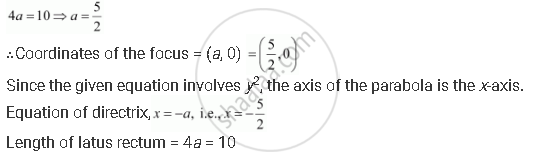CBSE (Arts) Class 11CBSE
Share
Notifications

View all notifications
Books Shortlist
Your shortlist is empty

# Find the Coordinates of the Focus, Axis of the Parabola, the Equation of Directrix and the Length of the Latus Rectum for Y2 = 10x - CBSE (Arts) Class 11 - Mathematics

Login
Create free account

Forgot password?
ConceptParabola Latus Rectum

#### Question

Find the coordinates of the focus, axis of the parabola, the equation of directrix and the length of the latus rectum for y2 = 10x

#### Solution

The given equation is y2 = 10x.

Here, the coefficient of is positive. Hence, the parabola opens towards the right.

On comparing this equation with y= 4ax, we obtainIs there an error in this question or solution?

#### APPEARS IN

Solution Find the Coordinates of the Focus, Axis of the Parabola, the Equation of Directrix and the Length of the Latus Rectum for Y2 = 10x Concept: Parabola - Latus Rectum.
S